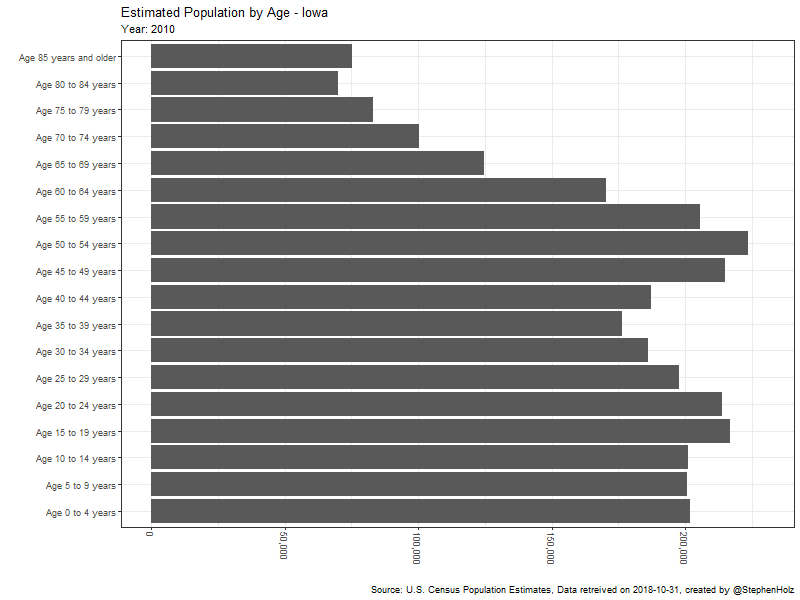The R code below can replicate this chart. You can get help installing R here.

You can get help with the tidycensus data retreival package here.

You can get help with the gganimate package here.

``````# Load R packages

library(tidycensus)
library(tidyverse)
library(gganimate)

# Get Data

population <- get_estimates(geography = 'state',
product = 'characteristics',
breakdown = c('SEX', 'AGEGROUP', 'HISP', 'RACE'),
state = 'IA',
breakdown_labels = TRUE,
time_series = TRUE)

# Wrangle Data

p <- population %>%
filter(
SEX == 'Both sexes',
str_detect(AGEGROUP, '^Age'),
HISP == 'Both Hispanic Origins',
RACE == 'All races',
DATE %in% c(3,10)
) %>%
mutate(
year = DATE + 2007
) %>%
ggplot() +
geom_bar(aes(x = AGEGROUP, y = value), stat = 'identity', position = 'identity') +
#facet_wrap(~RACE5,nrow = 2) +

scale_y_continuous(labels = scales::comma) +
coord_flip() +
theme_bw() +
theme(axis.text.x = element_text(angle = -90, hjust = 0, vjust = .5)) +
labs(
title = 'Estimated Population by Age - Iowa',
subtitle = 'Year: {closest_state}',
y = '',
x = '',
caption = paste0('Source: U.S. Census Population Estimates, Data retreived on ', Sys.Date(), ', created by @StephenHolz')
) +
transition_states(year, transition_length = 1, state_length = 2) +
ease_aes('linear')

animation <- animate(p,
width = 800,
height = 600
)

anim_save(
file.path('2017-IA-population-estimates-by-race-ethnicity-age.gif'),
animation = animation
)``````